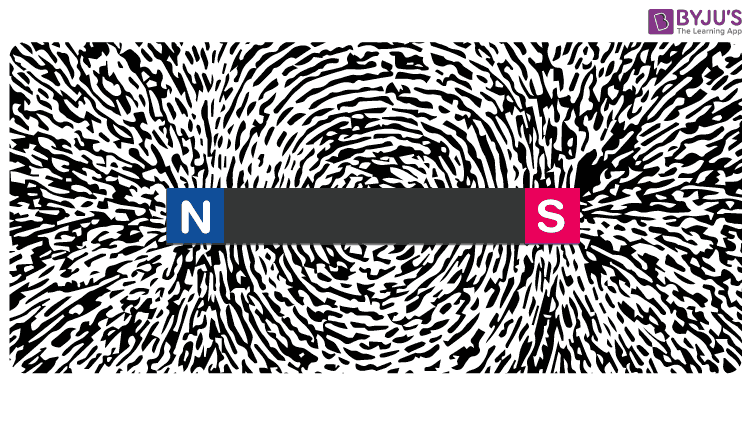# The Dipole in a Uniform Magnetic Field - Electrostatic Analog

In this section, we will learn about the nature of forces acting on a dipole placed in a uniform magnetic field and will compare it with the case when a dipole is kept in an electrostatic field. As we know that if we place iron filings around a bar magnet on a sheet of paper and tape the sheet, the fillings rearrange themselves to form a specific pattern. The pattern of iron filings here denotes the magnetic field lines generated due to the magnet. These magnetic field lines give us an approximate idea of the magnetic field B. But many times we are required to determine the magnitude of the magnetic field B accurately. We accomplish this by placing a small compass needle of known magnetic moment m and moment of inertia and allow it to oscillate in the magnetic field.The torque on the needle can be given as,

$$\begin{array}{l}\tau =m\times B\end{array}$$

The magnitude of this torque is given by mB sinƟ. Here τ is the restoring torque, and Ɵ is the angle between the direction of the magnetic moment (m) and the direction of the magnetic field (B).

At equilibrium, we can say that,

$$\begin{array}{l}I\frac{d^{2}\theta}{dt} \cong -mB\; sin\; \theta\end{array}$$

The negative sign in the above expression mB sinθ brings us to the conclusion that the restoring torque acting here acts in the opposite direction to the deflecting torque. Also, as the value of θ is very small in radians, we can approximate sin θ ≈ θ. Thus, using this approximation, we can write

$$\begin{array}{l}I\frac{d^{2}\theta}{dt} \cong -mB\; \theta\end{array}$$

Or,

$$\begin{array}{l}\frac{d^{2}\theta}{dt} \cong \frac{-mB\; \theta }{I}\end{array}$$

The above equation is a representation of a simple harmonic motion and the angular frequency can be given as,

$$\begin{array}{l}\omega =\frac{mB}{I}\end{array}$$

and thus, the time period can be stated as,

$$\begin{array}{l}T=2\pi \sqrt{\frac{I}{mB}}\end{array}$$

Or, we can also write it as,

$$\begin{array}{l}B=\frac{4\pi ^{2}I}{mT^{2}}\end{array}$$

The expression for magnetic potential energy is derived in the same manner as we derive the electrostatic potential energy as can be seen below. The magnetic potential energy Um can be given by

$$\begin{array}{l}U_{m}=\int \tau (\theta )d\theta \\ \\ \int mB\; sin\; \theta =-mB\; cos\; \theta =-m.B\end{array}$$

### Electrostatic Analog:

If we compare the equation derived above with the equations of electric dipole in an electric field, we conclude that the magnetic field due to a bar magnet at a large distance is analogous to that of an electric dipole in an electric field. The correspondence relation can be status as given below,

$$\begin{array}{l}E\rightarrow B,p\rightarrow m,\frac{1}{4\pi \epsilon _{0}}\rightarrow \frac{\mu _{0}}{4\pi }\end{array}$$

If the value of r, that is, the distance of the point from the given magnet is very large as compared to the size of the magnet given by I, or r >> l, then we can write the equatorial field generated by a bar magnet as,

$$\begin{array}{l}B_{E}=-\frac{\mu _{0}m}{4\pi r^{3}}\end{array}$$

Similarly, the axial field of the bar magnet in the same condition can be given as,

$$\begin{array}{l}B_{A}=-\frac{\mu _{0}2m}{4\pi r^{3}}\end{array}$$

Stay tuned with BYJU’S to learn more about dipole in a uniform magnetic field, the electrostatic analog, and other related topics.